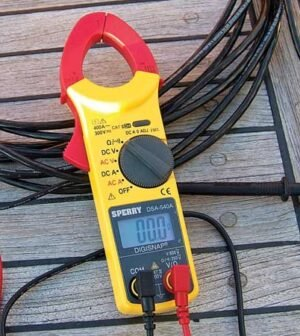# Electric Charge and Voltmeter

By on November 24, 2020What is an Electric Charge?

Electric charge is a basic physical property of a subatomic particle that causes it to experience a force when placed in an electric field or magnetic field. There are two types of charges, positive charge of the protons and negative charge of the electrons.

The electric charge is measured using the unit coulomb. One coulomb can be defined as the quantity of charge transferred in one second. The other units of electric charge are Faraday and Ampere-Hour.

Electrification

An atom as a whole is electrically neutral. This means that the atom contains an equal number of positively charged protons and negatively charged electrons. The neutrons present in the atom have zero charges. A body can be charged by the addition or removal of electrons. The process of charging a body is called electrification. The following are the three types of electrification

• Charging by friction
• Charging by conduction
• Charging by induction

Charging by friction is the process of transferring electrons from one body to another by rubbing two bodies against each other. The body which loses the electrons gets positively charged and the body which gains the electrons gets negatively charged. The two bodies must be insulators.

A good conductor can be charged by touching it with a charged body. This process is called charging by conduction. The nature of the charge acquired is the same as the charging body.

A body which is a good conductor can be charged by placing it next to a charged body. This process is called charging by induction. The nature of the induced charge is always opposite to the charging body.

#### Properties of Electric Charge

Some of the properties of charge are listed below

1. Like charges (positive-positive or negative-negative) will repel each other and unlike charges (positive-negative) attract each other.
2. Charged bodies attract light uncharged bodies.
3. Charges always lie on the outer surface of a conductor.
4. It is a scalar quantity.
5. The net charge of the system is equal to the sum of the charges comprising the system. The charge is additive in nature.
6. Charge of the body is velocity independent.
7. Accelerated charges produce electromagnetic waves.
8. The charge will not exist without mass.
9. The charge is conserved in an isolated system.
10. Charge is quantised. The charge “q” acquired by a body is an integral multiple of elementary charge “e”.

#### Coulombs law

Coulomb’s law helps us to calculate the strength of the forces between two charges. The law states that

“ The electrostatic force of attraction or repulsion between two stationary point charges is directly proportional to the product of the magnitudes of the charges and inversely proportional to the square of the distance between them”.

#### Voltmeter

The rate of flow of charge is called current. The current flow is measured using an instrument called an ammeter. The difference in charge between two points in a circuit is called voltage. The voltage is measured using a voltmeter. The voltmeter is connected parallel with the circuit. The resistance is infinity for an ideal voltmeter